The CUSUM Procedure

### Formulas for Cumulative Sums

#### One-Sided Cusum Schemes

##### Positive Shifts

If the shift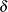to be detected is positive, the cusum computed for theth subgroup is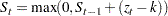for=1, 2, . . . ,, where=0,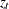is defined as for two-sided schemes, and the parameter, termed the reference value, is positive. The cusum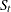is referred to as an upper cumulative sum. Sincecan be written as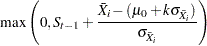the sequencecumulates deviations in the subgroup means greater thanstandard errors from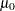. Ifexceeds a positive value(referred to as the decision interval), a shift or out-of-control condition is signaled. This formulation follows that of Lucas (1976), Lucas and Crosier (1982), and Montgomery (1996).

##### Negative Shifts

If the shiftto be detected is negative, the cusum computed for theth subgroup is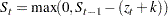for=1, 2, . . . ,, where=0,is defined as for two-sided cusum schemes, and the parameter, termed the reference value, is positive. The cusumis referred to as a lower cumulative sum. Sincecan be written as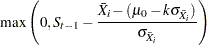the sequencecumulates the absolute value of deviations in the subgroup means less thanstandard errors from. Ifexceeds a positive value(referred to as the decision interval), a shift or out-of-control condition is signaled.

This formulation follows that of Lucas (1976), Lucas and Crosier (1982), and Montgomery (1996). Note thatis always positive andis always positive, regardless of whetheris positive or negative. For schemes designed to detect a negative shift, some authors, including van Dobben de Bruyn (1968) and Wadsworth, Stephens, and Godfrey (1986), define a reflected version offor which a shift is signaled whenis less than a negative limit.

Lucas and Crosier (1982) describe the properties of a fast initial response (FIR) feature for cusum schemes in which the initial cusumis set to a "headstart" value. Average run length calculations given by Lucas and Crosier (1982) show that the FIR feature has little effect when the process is in control and that it leads to a faster response to an initial out-of-control condition than a standard cusum scheme. You can provide headstart valuewith the HEADSTART= option or the variable _HSTART_ in a LIMITS= data set.

##### Constant Sample Sizes

When the subgroup sample sizes are constant (=), it may be preferable to compute cusums that are scaled in the same units as the data. Refer to Montgomery (1996) and Wadsworth, Stephens, and Godfrey (1986). To request this, specify the DATAUNITS option. Cusums are then computed as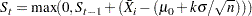for>0 and the equation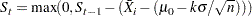for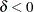. In either case, a shift is signaled ifexceeds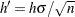. Wadsworth, Stephens, and Godfrey (1986) use the symbol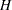for
.

If the subgroup sample sizes are not constant, you can specify a constant nominal sample sizewith the LIMITN= option or the variable _LIMITN_ in a LIMITS= data set. In this case, only those subgroups with sample sizeare analyzed unless you also specify the option ALLN. You can further specify the option NMARKERS to request special symbol markers for points corresponding to sample sizes not equal to.

#### Two-Sided Cusum Schemes

If the cusum scheme is two-sided, the cumulative sumplotted for theth subgroup is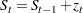for=1, 2, . . . ,. Here=0, and the termis calculated as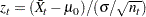where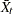is theth subgroup average, and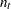is theth subgroup sample size. If the subgroup samples consist of individual measurements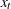, the termsimplifies to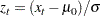Since the first equation can be rewritten as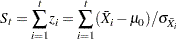the sequencecumulates standardized deviations of the subgroup averages from the target mean.

In many applications, the subgroup sample sizes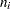are constant (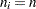), and the equation forcan be simplified.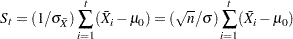In some applications, it may be preferable to computeas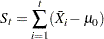which is scaled in the same units as the data. Refer to Montgomery (1996), Wadsworth, Stephens, and Godfrey (1986), and American Society for Quality Control (1983). If the subgroup sample sizes are constant (=) and if you specify the DATAUNITS option in the XCHART statement, the CUSUM procedure computes cusums using the final equation above. In this case, the procedure rescales the V-mask parametersandtoand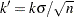, respectively. Wadsworth, Stephens, and Godfrey (1986) use the symbolsfor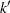andfor.

If the subgroup sample sizes are not constant, you can specify a constant nominal sample sizewith the LIMITN= option or with the variable _LIMITN_ in a LIMITS= data set. In this case, only those subgroups with sample sizeare analyzed unless you also specify the option ALLN. You can further specify the option NMARKERS to request special symbol markers for points corresponding to sample sizes not equal to.

If the process is in control and the meanis at or near the target, the points will not exhibit a trend since positive and negative displacements fromtend to cancel each other. Ifshifts in the positive direction, the points exhibit an upward trend, and ifshifts in the negative direction, the points exhibit a downward trend.Previous Page | Next Page | Top of Page